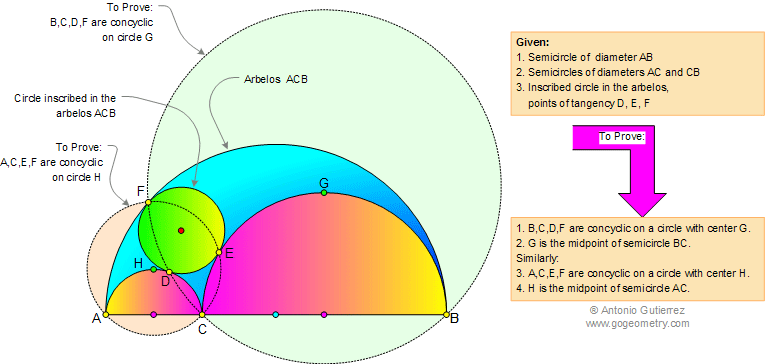Arbelos and Inscribed Circle: Concyclic Points. Level: High School, SAT Prep, CollegeGeometry Problem 638. If a circle is inscribed in the arbelos ACB with tangency points D, E, and F, as shown in the figure, then the points B, C, D, and F are concyclic on circle with center G and G is the midpoint of the semicircle of diameter BC. Similarly, A, C, E, and F are concyclic on circle with center H and H is the midpoint of semicircle of diameter AC.See Also: Geometry Problem 637 Semicircle, Diameter, Perpendicular, Inscribed Circle, Chord, Tangent, Arbelos. Geometry Problem 636 Semicircle, Diameter, Perpendicular, Inscribed Circle, Common Tangent.
 Home | Search | Geometry | Problems | All Problems | Visual Index | 631-640 | Arbelos | Tangent Circles | Circles | Semicircle | Perpendicular lines | Email | Post a comment or solution | By Antonio Gutierrez